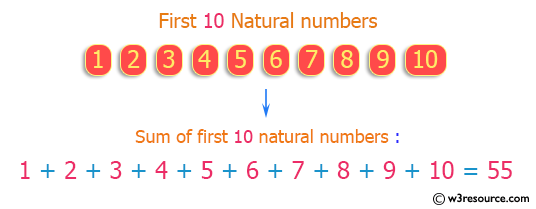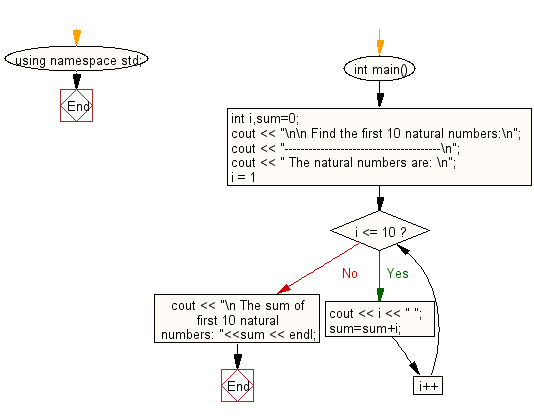﻿ C++ Exercises: Find the sum of first 10 natural numbers - w3resource

# C++ Exercises: Find the sum of first 10 natural numbers

## C++ For Loop: Exercise-2 with Solution

Write a program in C++ to find the sum of the first 10 natural numbers.

Pictorial Presentation:Sample Solution :-

C++ Code :

``````#include <iostream>
using namespace std;
int main()
{
int i,sum=0;
cout << "\n\n Find the first 10 natural numbers:\n";
cout << "---------------------------------------\n";
cout << " The natural numbers are: \n";
for (i = 1; i <= 10; i++)
{
cout << i << " ";
sum=sum+i;
}
cout << "\n The sum of first 10 natural numbers: "<<sum << endl;
}
``````

Sample Output:

``` Find the first 10 natural numbers:
---------------------------------------
The natural numbers are:
1 2 3 4 5 6 7 8 9 10
The sum of first 10 natural numbers: 55
```

Flowchart:C++ Code Editor: